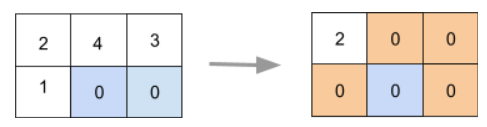4

# Zero Matrix

Difficulty: EASYContributed By
Deep Mavani
Avg. time to solve
20 min
Success Rate
80%

Problem Statement

You are given a matrix 'MATRIX' of dimension 'N' x 'M'. Your task is to make all the elements of row 'i' and column 'j' equal to 0 if any element in the ith row or jth column of the matrix is 0.

#### Note:

``````1) The number of rows should be at least 1.

2) The number of columns should be at least 1.

3) For example, refer to the below matrix illustration:
``````##### Input Format:
``````The first line of the input contains an integer 'T' denoting the number of test cases.

The first line of each test case contains two space-separated integers, 'N' and 'M', as described in the problem statement.

The next 'N' lines of each test case contain 'M' integers separated by spaces describing rows of the matrix.
``````
##### Output Format:
``````For each test case, return 'N' rows consisting of 'M' integers representing the matrix.
``````
##### Note:
``````You don't need to print anything, it has already been taken care of. Just implement the given function.
``````
##### Constraints:
``````1 <= T <= 50
1 <= N <= 100
1 <= M <= 100
-10^9 <= MATRIX[i][j] <= 10^9

Where 'MATRIX[i][j]' denotes the matrix element.
``````

``````Can you solve it with the space complexity of O(1)?

Time limit: 1 sec
``````
##### Sample Input 1:
``````2
2 3
2 4 3
1 0 0
2 2
0 1
2 3
``````
##### Sample Output 1:
``````2 0 0
0 0 0
0 0
0 3
``````
##### Explanation for Sample Output 1:
``````In test case 1, 2nd row, 2nd column, and 3rd column of the input matrix A contain 0. So, we have to make the entire 2nd row, 2nd column, and 3rd column of matrix A to 0. Aso explained in the above example with the matrix.

In test case 2, element at (0,0) has value 0 and so the entire row 1 and column 1 will be zero.
``````
##### Sample Input 2:
``````2
1 1
5
3 3
0 5 0
7 0 9
2 4 2
``````
##### Sample Output 2:
``````5
0 0 0
0 0 0
0 0 0
``````
##### Explanation for Sample Output 2:
``````In test case 1, the only element in the given matirx is 5 and so no 0's are involved.

In test case 2, 'MATRIX', 'MATRIX', and 'MATRIX' are 0 (0-based indexing are used). So, we have to make the entire 1st column, 2nd column, and 3rd column of matrix A to 0.
``````Console